吴恩达《神经网络和深度学习》课程笔记（2）-- 神经网络基础之逻辑回归1. Binary Classification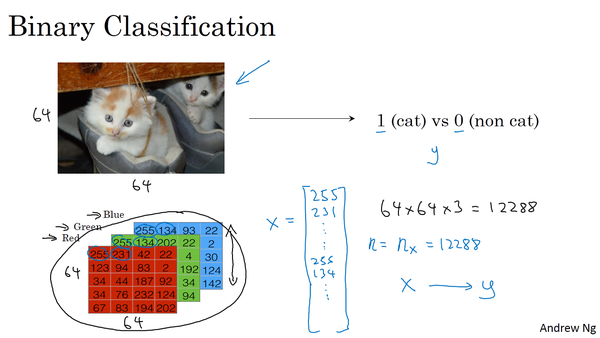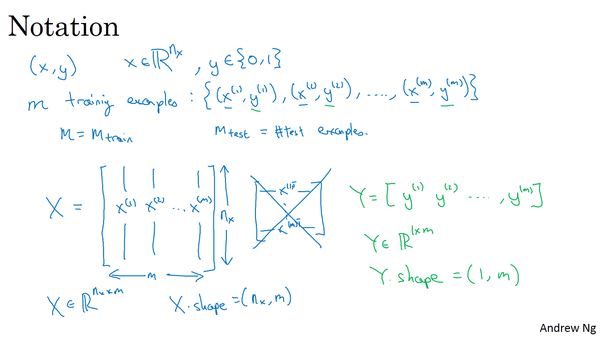Andrew还给出了本课程中关于深度学习一些常用的标准符号（notations），如下图所示。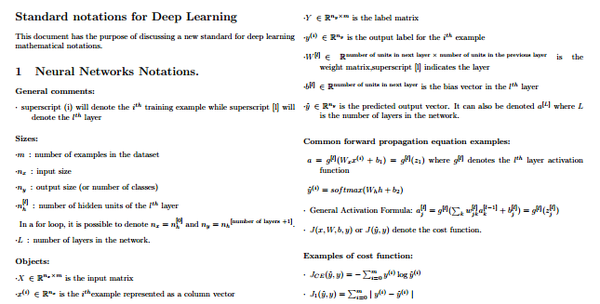2. Logistic RegressionSigmoid函数是一种非线性的S型函数，输出被限定在[0,1]之间，通常被用在神经网络中当作激活函数（Activation function）使用。Sigmoid函数的表达式和曲线如下所示：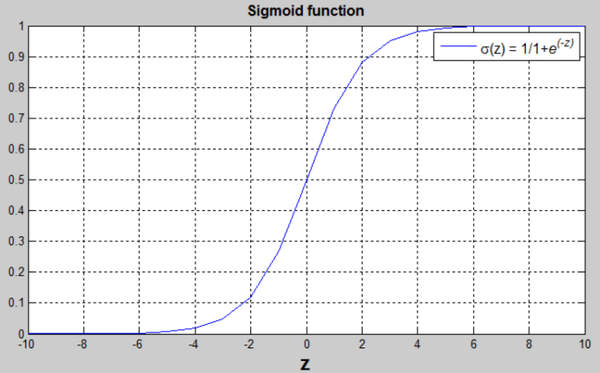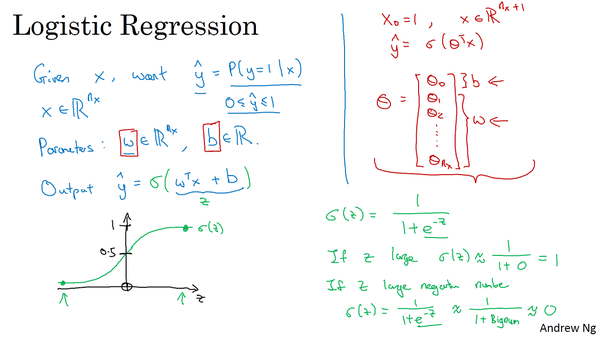3. Logistic Regression Cost FunctionLoss function的原则和目的就是要衡量预测输出 \hat y 与真实样本输出y的接近程度。平方错误其实也可以，只是它是non-convex的，不利于使用梯度下降算法来进行全局优化。因此，我们可以构建另外一种Loss function，且是convex的，如下所示：Cost function已经推导出来了，Cost function是关于待求系数w和b的函数。我们的目标就是迭代计算出最佳的w和b值，最小化Cost function，让Cost function尽可能地接近于零。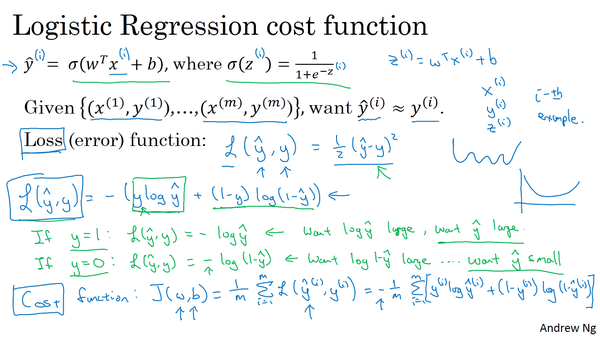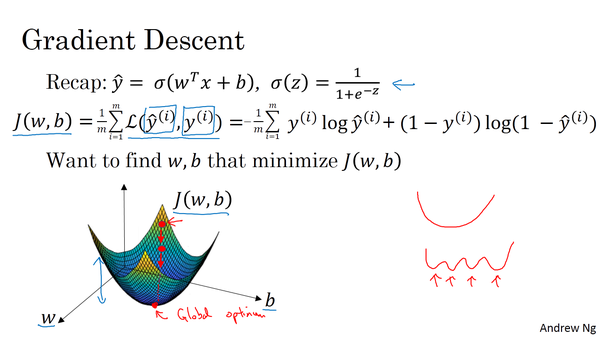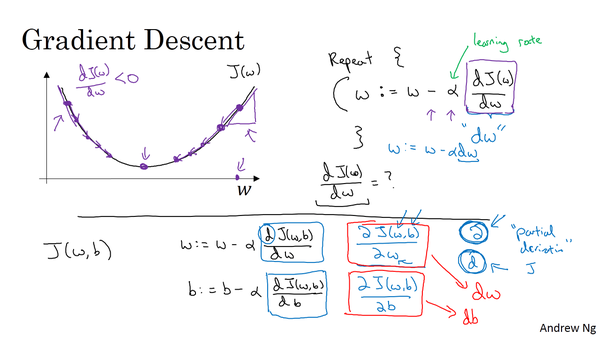5. Derivatives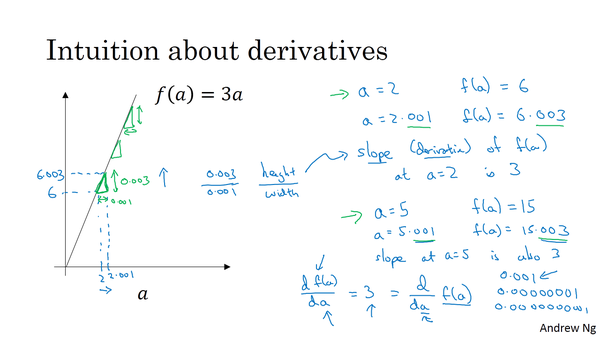6. More Derivative Examples

Andrew给出了更加复杂的求导数的例子：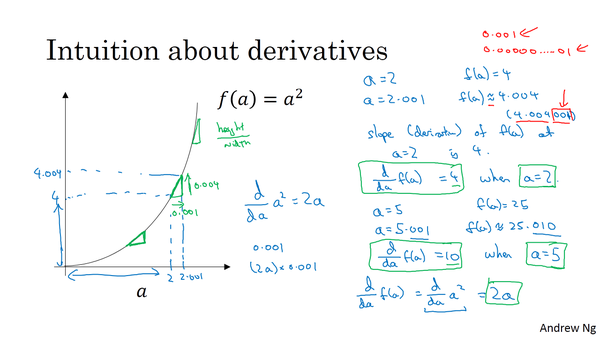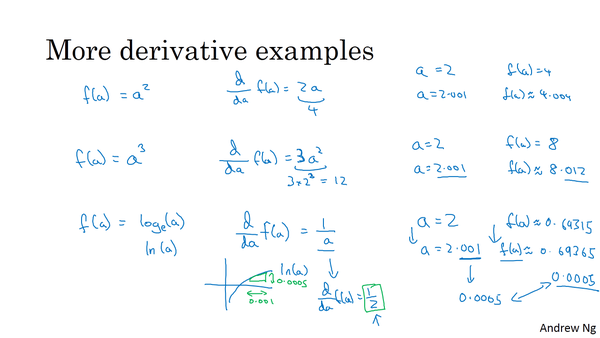7. Computation graph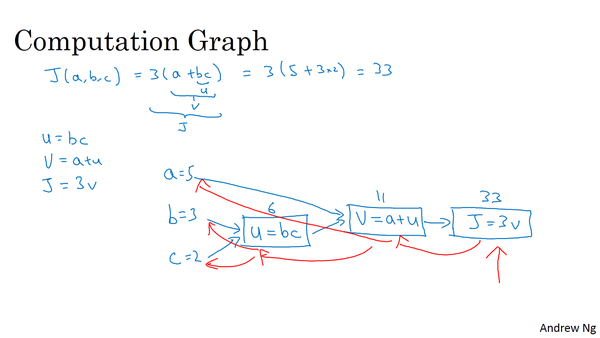8. Derivatives with a Computation Graph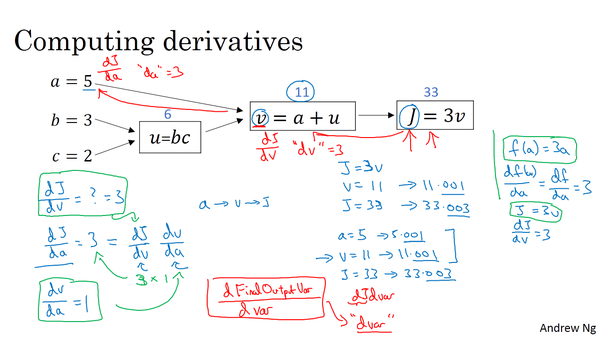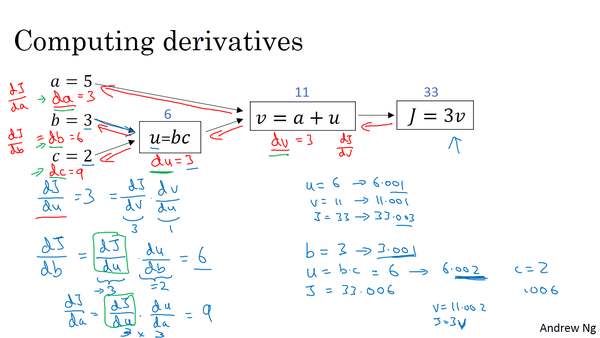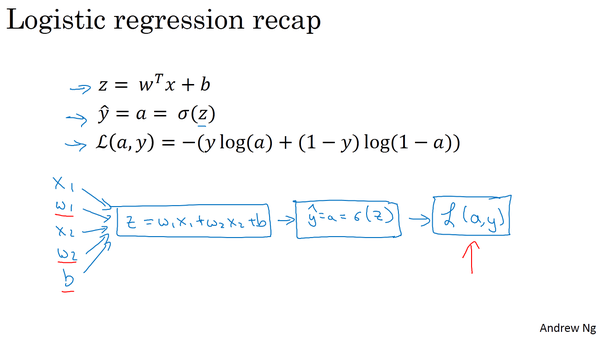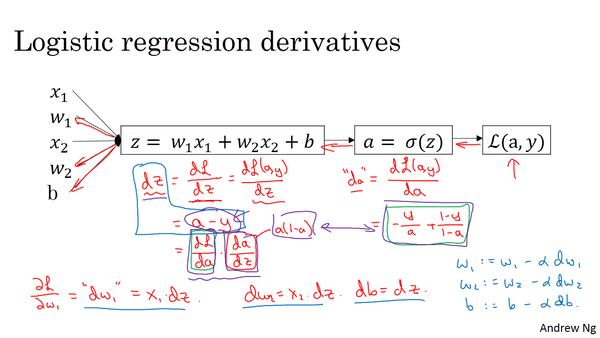10. Gradient descent on m examplesCost function关于w和b的偏导数可以写成和平均的形式：J=0; dw1=0; dw2=0; db=0;
for i = 1 to m
z(i) = wx(i)+b;
a(i) = sigmoid(z(i));
J += -[y(i)log(a(i))+(1-y(i)）log(1-a(i));
dz(i) = a(i)-y(i);
dw1 += x1(i)dz(i);
dw2 += x2(i)dz(i);
db += dz(i);
J /= m;
dw1 /= m;
dw2 /= m;
db /= m;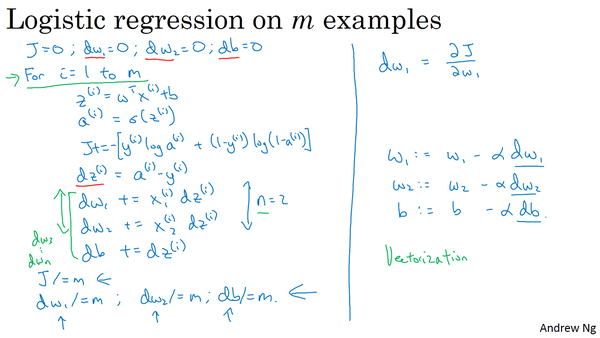Changelog

• 2017-09-24 创建